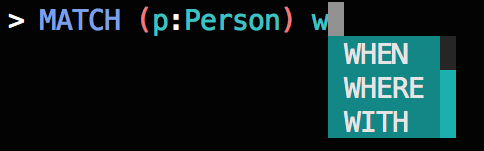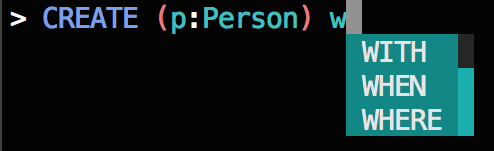Over a million developers have joined DZone.{{announcement.body}}
{{announcement.title}}

# Improving My CLI’s Autocomplete With Markov Chains

DZone 's Guide to

# Improving My CLI’s Autocomplete With Markov Chains

### How to get a list of autocompletions in CLI for Neo4j's Cypher query where the order of the keyword suggestions is tailored to the context of the query instead of listed in alphabetical order.

· Database Zone ·
Free Resource

Comment (0)

Save
{{ articles.views | formatCount}} Views

For a while I’ve been working on `cycli`, a command-line interface (CLI) for Neo4j‘s Cypher query language.

As demonstrated below, it autocompletes on your node labels, relationship types, property keys, and Cypher keywords. The autocompletion of the lattermost in this list–Cypher keywords–is the focus of this post.Cycli after the Markov chain update.

### The Problem

Originally, Cypher keywords were suggested simply in alphabetical order.

However, this was too naïve: If you typed a `W`, for example, the autocompletion menu would display the suggestions `WHEN``WHERE`, and `WITH` in that order, regardless of whether or not those keywords were valid or appropriate given the context of your query.These are no good:

• Exhibit A – `WHEN` is an invalid suggestion following a `MATCH` keyword.
• Exhibit B – Both `WHEN` and `WHERE` are invalid suggestions following a `CREATE` keyword.
• Exhibit C – This is the only example where the first keyword suggested is appropriate, but this is just by luck.

For the examples above, people who are familiar with Cypher can tell you that out of the Cypher keywords that start with `W`, the `WHERE`, and `WITH` keywords are most likely to follow the `MATCH `keyword, the `WITH `keyword is most likely to follow the `CREATE` keyword and the `WHEN` keyword is most likely to follow the `CASE` keyword.

For a better experience, typing a `W` should toggle an autocomplete menu where the order of the suggested keywords is a function of the most previous keyword in the query.

So how do I implement this?

I’m certainly not going to hardcode any of these rules into `cycli` manually; rather, I let the data speak for itself.

### The Solution

I scraped all Cypher queries from all of the GraphGists listed on the GraphGist wiki

GraphGists are what I like to call the IPython notebook of Cypher: They allow you to build a sort of notebook with inline Cypher queries, where the results are rendered in the browser. This provided a good sample dataset of Cypher queries for building the Markov chain.

As you might’ve read in a previous blog post of mine:

A Markov process consists of states and probabilities, where the probability of transitioning from one state to another depends only on the current state and not on the past; it is memoryless. A Markov process is often depicted as a transition probability matrix, where the(i,j)  entry of this matrix is the probability that the Markov process transitions to state j  given that the process is currently in state i.

We can apply this idea easily to Cypher queries by considering every Cypher keyword as a state in a Markov process.

In other words, if the last keyword you typed is `MATCH`, then you are currently in the `MATCH` state of the Markov process and there is a set of probabilities indicating which states, or Cypher keywords, you will most likely enter / use next.

The solution then is to build a transition probability matrix with our sample dataset of Cypher queries.

### Methodology

For the sake of demonstration, let’s pretend the following Cypher queries comprise our sample dataset. Let’s also pretend that Cypher keywords consist only of the keywords within these queries.

``````keywords = ["CREATE", "RETURN", "MATCH", "WHERE", "WITH"]

queries = [
"MATCH n WHERE n.name = 'Nicole' WITH n RETURN n;",
"MATCH n WHERE n.age > 50 RETURN n;",
"MATCH n WITH n RETURN n.age;",
"CREATE n RETURN n;",
]``````

I decided to initially store the Markov process in a dictionary of dictionaries so that the probabilities can be accessed with `markov["i"]["j"]`, which is the probability that the next keyword is j given that the current keyword is i.

I’ve included an additional state—the empty state (represented by an empty string)—to also cover the case where there are no previous keywords, as we still want to recommend keywords ordered by the most probable even for the first keyword in the query.

First, I build the initial data structure.

``````states = [""] + keywords
markov = {i: {j:0.0 for j in states} for i in states}

print(markov)``````
``````{
"": {
"": 0.0,
"CREATE": 0.0,
"MATCH": 0.0,
"RETURN": 0.0,
"WHERE": 0.0,
"WITH": 0.0
},
"CREATE": {
"": 0.0,
"CREATE": 0.0,
"MATCH": 0.0,
"RETURN": 0.0,
"WHERE": 0.0,
"WITH": 0.0
},
"MATCH": {
"": 0.0,
"CREATE": 0.0,
"MATCH": 0.0,
"RETURN": 0.0,
"WHERE": 0.0,
"WITH": 0.0
},
"RETURN": {
"": 0.0,
"CREATE": 0.0,
"MATCH": 0.0,
"RETURN": 0.0,
"WHERE": 0.0,
"WITH": 0.0
},
"WHERE": {
"": 0.0,
"CREATE": 0.0,
"MATCH": 0.0,
"RETURN": 0.0,
"WHERE": 0.0,
"WITH": 0.0
},
"WITH": {
"": 0.0,
"CREATE": 0.0,
"MATCH": 0.0,
"RETURN": 0.0,
"WHERE": 0.0,
"WITH": 0.0
}
}``````

Next, for each query, I find the positions of each keyword and order the keywords by these positions ascending.

Then I iterate through this list of keywords and increment the `markov["i"]["j"]` value by 1 each time I see keyword j follow keyword i.

Note that the actual implementation is a bit beefier, as it takes care to not include words that are node labels, relationship types, strings, etc.

``````import re

for query in queries:
# Find the positions of Cypher keywords.
positions = []

for word in keywords:
idx = [m.start() for m in re.finditer(word, query)]

for i in idx:
positions.append((word, i))

# Sort the words by the order of their positions in the query.
positions.sort(key=lambda x: x)

# Drop the indexes.
positions = [x for x in positions]

# Prepend the empty state to the list of keywords.
positions = [""] + positions

# Build the Markov model.
for i in range(len(positions) - 1):
current_keyword = positions[i]
next_keyword = positions[i + 1]

markov[current_keyword][next_keyword] += 1

print(markov)``````
``````{
"": {
"": 0.0,
"CREATE": 1.0,
"MATCH": 3.0,
"RETURN": 0.0,
"WHERE": 0.0,
"WITH": 0.0
},
"CREATE": {
"": 0.0,
"CREATE": 0.0,
"MATCH": 0.0,
"RETURN": 1.0,
"WHERE": 0.0,
"WITH": 0.0
},
"MATCH": {
"": 0.0,
"CREATE": 0.0,
"MATCH": 0.0,
"RETURN": 0.0,
"WHERE": 2.0,
"WITH": 1.0
},
"RETURN": {
"": 0.0,
"CREATE": 0.0,
"MATCH": 0.0,
"RETURN": 0.0,
"WHERE": 0.0,
"WITH": 0.0
},
"WHERE": {
"": 0.0,
"CREATE": 0.0,
"MATCH": 0.0,
"RETURN": 1.0,
"WHERE": 0.0,
"WITH": 1.0
},
"WITH": {
"": 0.0,
"CREATE": 0.0,
"MATCH": 0.0,
"RETURN": 2.0,
"WHERE": 0.0,
"WITH": 0.0
}
}``````

Next, I divide each value in each row by the sum of the row so that I have probabilities.

``````for word, states in markov.items():
denominator = sum(states.values())

if denominator == 0:
# Absorbing state.
markov[word] = {i: 1.0 if i == word else 0.0 for i in states.keys()}
elif denominator > 0:
markov[word] = {i:j / denominator for i, j in states.items()}

print(markov)``````
``````{
"": {
"": 0.0,
"CREATE": 0.25,
"MATCH": 0.75,
"RETURN": 0.0,
"WHERE": 0.0,
"WITH": 0.0
},
"CREATE": {
"": 0.0,
"CREATE": 0.0,
"MATCH": 0.0,
"RETURN": 1.0,
"WHERE": 0.0,
"WITH": 0.0
},
"MATCH": {
"": 0.0,
"CREATE": 0.0,
"MATCH": 0.0,
"RETURN": 0.0,
"WHERE": 0.6666666666666666,
"WITH": 0.3333333333333333
},
"RETURN": {
"": 0.0,
"CREATE": 0.0,
"MATCH": 0.0,
"RETURN": 1.0,
"WHERE": 0.0,
"WITH": 0.0
},
"WHERE": {
"": 0.0,
"CREATE": 0.0,
"MATCH": 0.0,
"RETURN": 0.5,
"WHERE": 0.0,
"WITH": 0.5
},
"WITH": {
"": 0.0,
"CREATE": 0.0,
"MATCH": 0.0,
"RETURN": 1.0,
"WHERE": 0.0,
"WITH": 0.0
}
}``````

The probabilities in each row now sum to 1.

Finally, I convert the dictionaries to a list of tuples so that they can be maintained in order of probability descending (dictionaries have no sense of order).

``````for word in markov.keys():
ordered = sorted(markov[word].items(), key=lambda x:x, reverse=True)
markov[word] = ordered``````

Now, I have a data structure that can tell me which keywords are most likely to be used next given the current keyword.

For example, with this pretend dataset, if the current keyword is `MATCH`, then the probability of the next keyword being `WHERE` is 67% and the probability of the next keyword being `WITH` is 33%:

``````for state in markov["MATCH"]:
print(state)``````
``````('WHERE', 0.6666666666666666)
('WITH', 0.3333333333333333)
('', 0.0)
('RETURN', 0.0)
('CREATE', 0.0)
('MATCH', 0.0)``````

### Ship It!

This workflow was applied to the full sample of Cypher queries scraped from the GraphGists wiki and the resulting data structure – the dictionary of tuples – is now included in `cycli` to make smarter autocomplete suggestions for Cypher keywords.

Let’s look at the real data for a few keywords.

``from cycli.markov import markov``

### MATCH

If your most recent keyword is `MATCH`:

``````for state in markov["MATCH"][:5]:
print(state)``````
``````('CREATE', 0.7143900657414171)
('MATCH', 0.10689067445824203)
('WHERE', 0.0723155588020453)
('RETURN', 0.0577063550036523)
('WITH', 0.01996591185780375)``````

### CREATE

If your most recent keyword is `CREATE`:

``````for state in markov["CREATE"][:5]:
print(state)``````
``````('CREATE', 0.7466683589287981)
('UNIQUE', 0.24152811270465796)
('RETURN', 0.002792232516816855)
('DELETE', 0.002284553877395609)
('INDEX', 0.0020307145576849853)``````

### COUNT

If your most recent keyword is `COUNT`:

``````for state in markov["COUNT"][:5]:
print(state)``````
``````('AS', 0.875)
('COLLECT', 0.07142857142857142)
('DESC', 0.017857142857142856)
('ORDER', 0.017857142857142856)
('ASC', 0.017857142857142856)``````

### The Results

Revisiting the examples from earlier, where typing a `W` in previous versions of `cycli` yielded an alphabetical list of Cypher keywords with no regard to whether or not they were valid or appropriate, we now get a list of autocompletions where the order of the keyword suggestions is tailored to the context of the query.Much better:

• Exhibit A – `WHERE` and `WITH` are appropriate suggestions to follow a `MATCH` keyword and are ordered correctly, as `WHERE` is more likely to follow than `WITH`.
• Exhibit B – In the case of the `CREATE` keyword, `WITH` is the best suggestion as both `WHEN` and`WHERE` are invalid.
• Exhibit C – `WHEN` is the only valid suggestion for a keyword following the `CASE` keyword.

This is easily accomplished behind the scenes with the `markov` chain data structure now included in `cycli`.

### Exhibit A

``````for word, probability in markov["MATCH"]:
if word.startswith("W"):
print(word, probability)``````
``````WHERE 0.0723155588020453
WITH 0.01996591185780375
WHEN 0.0``````

### Exhibit B

``````for word, probability in markov["CREATE"]:
if word.startswith("W"):
print(word, probability)``````
``````WITH 0.0013961162584084274
WHEN 0.0
WHERE 0.0``````

### Exhibit C

``````for word, probability in markov["CASE"]:
if word.startswith("W"):
print(word, probability)``````
``````WHEN 0.9666666666666667
WITH 0.0
WHERE 0.0``````

### Next Steps

While `cycli` is only utilizing the one-step probabilities in this Markov process, I wanted to build this data structure to open the door for some fun, offline analysis of the Cypher query language.

With a Markov chain, I can answer questions like:

• What is the steady state distribution of a Cypher query?
• What is the expected number of steps until a user reaches the `COLLECT` state?
• Given that a user is currently in the `MATCH` state, what is the expected number of steps until the user returns to the `MATCH` state?

I plan to answer these types of questions in a future post.

This article was written by Nicole White.

[This post was originally published on Nicole White’s blog and was used with permission.]

Topics:
cli ,markov chain ,cycli ,neo4j ,database ,cypher

Comment (0)

Save
{{ articles.views | formatCount}} Views

Published at DZone with permission of

Opinions expressed by DZone contributors are their own.

# {{ parent.title || parent.header.title}}

{{ parent.tldr }}

{{ parent.urlSource.name }}